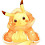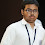### Constructions in Number Theory

Hi, I am Emon, a ninth grader, an olympiad aspirant, hailing from Kolkata. I love to do olympiad maths and do some competitive programming in my leisure hours, though I take it seriously. I had written INOI this year.

Today, I would be giving you a few ideas on how to Construct in Number Theory. Well, so we shall start from the basics and shall try to dig deeper into it with the help of some important and well-known theorems and perhaps, some fancy ones as well. Okay, so without further delay, let's start off...

What do we mean by "Constructions"?

If noticed, you can see that you often face with some problems in olympiad saying, "... Prove that there exists infinitely many numbers satisfying the given conditions" or "... Prove that there exists a number satisfying the above conditions." These are usually the construction-problems

For example, let's consider a trivial example :

Problem. Prove that there exist infinitely many integers $a$ such that $3^a+1$ is divisible by $4$.

Solution. This is a one-liner. Take $a$ as any odd number greater than or equal to $1$. Then, since $x+y\mid x^n+y^n$ if $n$ is an odd number, we have $4\mid 3^a+1$, as desired.

I hope you have got a basic idea on how to construct.

The Chinese Remainder Theorem

Many among you might know the Chinese Remainder. Actually, it all started with a problem with specific numbers in a book called Sun-Tzu Suan-ching written by chinese mathematician Sun-Tzu.

Theorem. Let $n_1, n_2, \ldots, n_r$ be a set of pairwise coprime integers. Let $(a_1, a_2,\cdots, a_r)\in \mathbb{N}$. Then, there exists a unique $x\in \{1, 2, \cdots, n_1n_2\cdots n_r\}$ such that \begin{aligned} x &\equiv a_1\pmod{n_1} \\ x &\equiv a_2\pmod{n_2} \\ &\ \ \vdots \\ x &\equiv a_r\pmod {n_r}. \end{aligned}

$Proof.$ Let $N=\prod_{i=1}^rn_i$. For each $k=1, 2, \ldots, r$, let

\begin{aligned} N_k &= \frac{N}{n_k} \\ &= n_1n_2\cdots n_{k-1}n_{k+1}\cdots n_r. \end{aligned}

Now, the $n_i'$s are relatively prime in pairs, so that $\gcd(N_k, n_k)=1$. Hence, by the Theory of Single Linear Congruence, the congruence $N_kx\equiv 1\pmod{n_k}$ is solvable. Let the unique solution be called $x_k$.

Claim. $y=\sum_{i=1}^ra_iN_ix_i$ is a simultaneous solution to the given system of congruences.

$Proof.$ We first observe that $N_i\equiv 0\pmod{n_j}\ \forall\ j\ne i$. Then, we have, \begin{aligned} y=\sum_{i=1}^ra_iN_ix_i &\equiv 0+0+\ldots+0+a_kN_kx_k+0+\ldots+0 \\ &\equiv a_kN_kx_k\pmod{n_k}. \end{aligned}

But, $x_k$ was chosen to satisfy the congruence $N_kx\equiv 1\pmod{n_k}$, implying that $a_kN_kx_k\equiv a_k\pmod{n_k}$, implying that there exists a solution to given system of congruences.

But, it still remains to show that the solution is a unique one. Suppose, for the sake of contradiction, there exists another integer $x_0$ such that $x_0\equiv y\pmod{n_k}$. Hence, $y-x_0\equiv 0\pmod{n_k}$ for each value of $k$. Now, since $\gcd(n_i, n_j)=1$, we have, $n_1\cdots n_r\mid y-x_0$, implying that $y\equiv x_0\pmod{N}$. Since $y\le N$, therefore, $x_0\ge N$ which implies that either $x_0=y$ or $x_0>N$, thus proving it's uniqueness.

Thue's Lemma

Thue’s lemma is a wonderful result in modular arithmetic, and is very useful in constructions, especially related to squares.

Lemma. Let $n> 1$ be an integer and $a$ be an integer coprime to it. Then there exists integers $k, \ell$ with $0< |k|, |\ell|<\sqrt{n}$ such that  $$ak\equiv \ell\pmod{n}.$$

$Proof.$ Let $m=\lfloor \sqrt{n}\rfloor$. Then $m^2\le n< (m+1)^2$. Now, we consider the numbers of the form $ax-y\ \forall\ 0\le x, y \le m$. There are $(m+1)^2>n$ such numbers. Hence, by PHP, atleast two are the same. So, for some $k_1, \ell_1, k_2, \ell_2$, we have, \begin{aligned} ak_1 -\ell_1 &\equiv ak_2-\ell_2 \pmod{n} \\ \iff a(k_1-k_2) &\equiv \ell_1-ell_2\pmod{n}. \end{aligned}

Setting $k=k_1-k_2$ and $\ell=\ell_1-\ell_2$, we get,  $$ak\equiv \ell\pmod{n}\text{, as desired}.$$

Fermat's Two-Square Theorem

Albert Girard was the first to make the observation that every prime $p$ of the form $4n+1$ is the sum of two squares is sometimes called Girard's theoremFermat wrote an elaborate version of the statement (in which he also gave the number of possible expressions of the powers of p as a sum of two squares) in a letter to Marin Mersenne dated December $25$, $1640$ : for this reason this version of the theorem is sometimes called Fermat's Christmas theorem.

Theorem. Let $p$ be an odd prime. Then there exist integers $x, y$ such that $p = x^2 + y^2$ if and only if $p \equiv 1 \pmod 4$.

$Proof.$ We first recall what Fermat's Christmas Theorem stated. According to it, there exists $x$ such that $p \mid x^2+1$ if and only if $p \equiv 1 \pmod 4$.

Now, suppose we have a number $q$ coprime to $p$. Then, by Thue's Lemma, we can find $x$ and $y$ such that $0<|x|, |y|<\sqrt{p}$ with $qy\equiv x\pmod p$. Hence, we have, $p\mid x^2-q^2y^2$. Next, we choose $q$ such that $q^2\equiv -1\pmod p$. We can do this due to FCT. Then, we get, $p\mid x^2+y^2$. But, we observe that $0<x^2+y^2<p+p=2p$ and hence $x^2+y^2=p$ as their is no other multiple of $p$ in between $0$ and $2p$.

Interesting Fact. The Fermat's Two-square theorem can be proved using Thue's Lemma, and on the other hand, Thue's Lemma can be proved using Fermat's Two-square theorem.

We now only state two theorems, one of them being a fancy one.

Lagrange Interpolation

Actually, the method of ''Lagrange Interpolation" was first discovered by Edward Waring in the year $1779$, but as can be understood, did not publish it. Later on, in $1795$, it was published by Joseph-Louis Lagrange. It is also an easy consequence of a formula published in $1783$ by Leonhard Euler.

Theorem. If $x_1,x_2,\ldots , x_n$ be $n$ distinct complex numbers and $a_1,a_2,\ldots, a_{n}$ be $n$ complex numbers, then there exists a unique polynomial $P$ of degree less than or equal to $n$ such that $P(x_i)=a_i\ \forall\ i.$ Further, if $P(x_i)=a_i$, then $$P(x)=\displaystyle{\sum_{i=0}^na_i\prod_{0\le i\ne j\le n}\frac{x-x_j}{x_i-x_j}}.$$

Pell's Equation

This equation was first studied extensively in India starting with Brahmagupta who found an integer solution to $92x^2+1=y^2$ in his Brahmasphutasiddhanta circa $628$. The Pell's Equation is also known as the Pell-Fermat Equation.

Theorem. The Pell's Equation $x^2-dy^2=1$ where $d$ is a square-free integer has infinitely many solutions $(x, y)$.

Solved Examples

Example $1$ (All Russian MO $2018$ Grade $10/6$). Let $a$ and $b$ be given positive integers. Prove that there are infinitely many positive integers $n$ such that $n^b + 1$ doesn’t divide $a^n + 1$.

Solution. We first choose a prime $p$ dividing $n^b+1$ that doesn't divide $a^n+1$. So, we want to construct a number $n$ such that $p\mid n^b+1$. Hence, $$n^b\equiv -1\pmod p.$$ So, we want an $n$ such that $\mathrm{ord}_p(n)=2b$. Let $p\nmid q$. Then, \begin{aligned} n^{2b} &\equiv q^{p-1}\pmod p \\ \iff n &\equiv q^{\frac{p-1}{2b}}\pmod p. \end{aligned} So, we want to get that $2b\mid p-1$. Next, we want $p\nmid a^n+1$ or $a^n+1\not\equiv 0\pmod p.$ We do one thing, we take $n$ such that $p-1\mid n$.  Let $n=(p-1)r$ for some $r$. Then, $$a^n+1=a^{(p-1)r}+ 1 \equiv 1+1=2\not \equiv 0\pmod p.$$ Thus, we make the following constructions \begin{aligned} \begin{cases} n &\equiv q^{\frac{p-1}{2b}}\pmod p \\ n &\equiv 0\pmod{p-1}\\ p &\equiv 1\pmod{2b}. \end{cases} \end{aligned} Now, we can choose infinitely many primes $p$ with $p\equiv 1\pmod{2b}$ due to Dirichlet's Theorem on Arithmetic Progression. Hence, we can conclude that there exists infinitely many such $n'$s.

Example $2$ (RMM $2012/4$). Prove that there are infinitely many positive integers $n$ such that $2^{2^n+1}+1$ is divisible by $n$ but $2^n+1$ is not.

Solution.  We first claim that if a number $k$ satisfies the conditions, then so does $\ell=2^k+1>k$.

Claim. If $k$ satisfies the conditions, then so does $2^k+1$.

$Proof.$ Let $2^k+1=\ell>k$. Firstly, since $\ell$ is not divisible by $k$, therefore, $2^{\ell}+1$ is not divisible by $2^k+1$. Hence, $\ell \nmid 2^{\ell}+1$. But, note that $$2^{2^{2^k+1}+1}+1 = (2^k+1)(\text{something}),$$ since $k\mid 2^{2^k+1}+1$. Hence, $2^k+1\mid 2^{2^{2^k+1}+1}+1$, implying that $\ell\mid 2^{2^\ell+1}+1.$ So, $\ell\mid 2^{2^\ell+1}+1$ and $\ell\nmid 2^\ell+1$, as desired. Now, some checking, starting from $5\times 3$, $7\times 3$, $11\times 3,\ldots,$ $19\times 3$, we see that at last $k=19\times 3=57$ works!

Hence, we can conclude that there exists infinitely many such positive integers $n$ satisfying the given conditions.

Okay, so that's enough, I guess... If you want to look into or try more problems, you can get them here. If you have have questions regarding this post, please feel free to comment. Also, if there is a mistake in this post, please do comment. I shall try to correct it. Hope to see you soon. Until then, bye...

Emon.

1.Very nicely written!

1.Thank you, Sunaina.

2.Nice work, i appreciate your effort for making a 25-problem pset, I think this is some unique and hard work by you.

### The importance of "intuition" in geometry

Hii everyone! Today I will be discussing a few geometry problems in which once you "guess" or "claim" the important things, then the problem can easily be finished using not-so-fancy techniques (e.g. angle chasing, power-of-point etc. Sometimes you would want to use inversion or projective geometry but once you have figured out that some particular synthetic property should hold, the finish shouldn't be that non trivial) This post stresses more about intuition rather than being rigorous. When I did these problems myself, I used freehand diagrams (not geogebra or ruler/compass) because I feel that gives a lot more freedom to you. By freedom, I mean, the power to guess. To elaborate on this - Suppose you drew a perfect  diagram on paper using ruler and compass, then you would be too rigid on what is true in the diagram which you drew. But sometimes that might just be a coincidence. e.g. Let's say a question says $D$ is a random point on segment $BC$, so maybe

### LMAO Revenge

Continuing the tradition of past years, our seniors at the Indian IMO camp(an unofficial one happened this year) once again conducted LMAO, essentially ELMO but Indian. Sadly, only those who were in the unofficial IMOTC conducted by Pranav, Atul, Sunaina, Gunjan and others could participate in that. We all were super excited for the problems but I ended up not really trying the problems because of school things and stuff yet I solved problem 1 or so did I think. Problem 1:  There is a   grid of real numbers. In a move, you can pick any real number  ,  and any row or column and replace every entry   in it with  .  Is it possible to reach any grid from any other by a finite sequence of such moves? It turned out that I fakesolved and oh my god I was so disgusted, no way this proof could be false and then when I was asked Atul, it turns out that even my answer was wrong and he didn't even read the proof, this made me even more angry and guess what? I was not alone, Krutarth too fakesol

### Edge querying in graph theory

In this post, I will present three graph theory problems in increasing difficulty, each with a common theme that one would determine a property of an edge in a complete graph through repeated iterations, and seek to achieve a greater objective. ESPR Summer Program Application: Alice and Bob play the following game on a $K_n$ ($n\ge 3$): initially all edges are uncolored, and each turn, Alice chooses an uncolored edge then Bob chooses to color it red or blue. The game ends when any vertex is adjacent to $n-1$ red edges, or when every edge is colored; Bob wins if and only if both condition holds at that time. Devise a winning strategy for Bob. This is more of a warm-up to the post, since it has a different flavor from the other two problems, and isn't as demanding in terms of experience with combinatorics. However, do note that when this problem was first presented, applicants did not know the winner ahead of time; it would be difficult to believe that Bob can hold such a strong